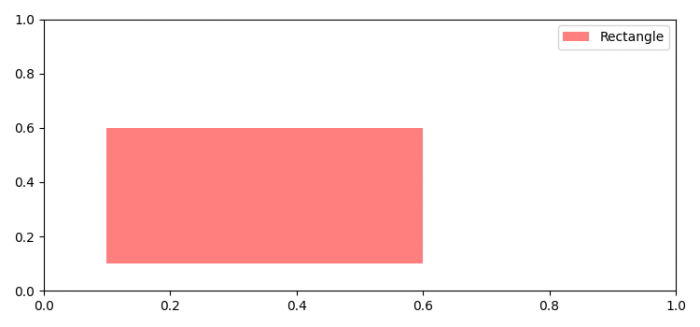# How to label a patch in matplotlib?

To label a patch in matplotlib, we can take the following steps −

• Set the figure size and adjust the padding between and around the subplots.

• Initialize the center of the rectangle patch.

• Create a new figure or activate an existing figure.

• Add an 'ax' to the figure as part of a subplot arrangement.

• Add a 'rectangle' to the axes' patches; return the patch.

• Place a legend on the figure.

• To display the figure, use show() method.

## Example

import matplotlib.pyplot as plt
import matplotlib.patches as patches

plt.rcParams["figure.figsize"] = [7.50, 3.50]
plt.rcParams["figure.autolayout"] = True

x = y = 0.1
fig = plt.figure()
plt.show()